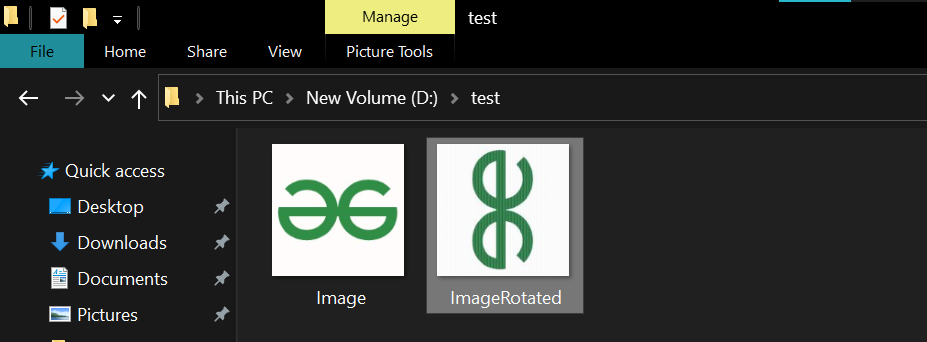# Java Program to Rotate an Image

• Last Updated : 25 Nov, 2021

The problem statement is to rotate an image clockwise 90 degrees for which here we will be using some in-built methods of BufferedImage class and Color c

Classes required to perform the operation is as follows:

• To read and write an image file we have to import the File class. This class represents file and directory path names in general.
• To handle errors we use the IOException class.
• To hold the image we create the BufferedImage object for that we use BufferedImage class. This object is used to store an image in RAM.
• To perform the image read-write operation we will import the ImageIO class. This class has static methods to read and write an image.
• This Graphics2D class extends the Graphics class to provide more sophisticated control over geometry, coordinate transformations, color management, and text layout. This is the fundamental class for rendering 2-dimensional shapes, text, and images on the Java(tm) platform.

Example:

## Java

 `// Java program to rotate image by 90 degrees clockwise` `// Importing classes from java.awt package for``// painting graphics and images``import` `java.awt.Graphics2D;``import` `java.awt.image.BufferedImage;``// Importing input output classes``import` `java.io.File;``import` `java.io.IOException;``import` `javax.imageio.ImageIO;` `// Main class``public` `class` `GFG {` `    ``// Method 1``    ``// To return rotated image``    ``public` `static` `BufferedImage rotate(BufferedImage img)``    ``{` `        ``// Getting Dimensions of image``        ``int` `width = img.getWidth();``        ``int` `height = img.getHeight();` `        ``// Creating a new buffered image``        ``BufferedImage newImage = ``new` `BufferedImage(``            ``img.getWidth(), img.getHeight(), img.getType());` `        ``// creating Graphics in buffered image``        ``Graphics2D g2 = newImage.createGraphics();` `        ``// Rotating image by degrees using toradians()``        ``// method``        ``// and setting new dimension t it``        ``g2.rotate(Math.toRadians(``90``), width / ``2``,``                  ``height / ``2``);``        ``g2.drawImage(img, ``null``, ``0``, ``0``);` `        ``// Return rotated buffer image``        ``return` `newImage;``    ``}` `    ``// Method 2``    ``// Main driver method``    ``public` `static` `void` `main(String[] args)``    ``{``        ``// try block to check for exceptions``        ``try` `{` `            ``// Reading original image``            ``BufferedImage originalImg = ImageIO.read(``                ``new` `File(``"D:/test/Image.jpeg"``));` `            ``// Getting and Printing dimensions of original``            ``// image``            ``System.out.println(``"Original Image Dimension: "``                               ``+ originalImg.getWidth() + ``"x"``                               ``+ originalImg.getHeight());` `            ``// Creating a subimage of given dimensions``            ``BufferedImage SubImg = rotate(originalImg);` `            ``// Printing Dimensions of new image created``            ``// (Rotated image)``            ``System.out.println(``"Cropped Image Dimension: "``                               ``+ SubImg.getWidth() + ``"x"``                               ``+ SubImg.getHeight());` `            ``// Creating new file for rotated image``            ``File outputfile``                ``= ``new` `File(``"D:/test/ImageRotated.jpeg"``);` `            ``// Writing image in new file created``            ``ImageIO.write(SubImg, ``"jpg"``, outputfile);` `            ``// Printing executed message``            ``System.out.println(``                ``"Image rotated successfully: "``                ``+ outputfile.getPath());``        ``}` `        ``// Catch block to handle the exception``        ``catch` `(IOException e) {` `            ``// Print the line number where exception``            ``// occurred``            ``e.printStackTrace();``        ``}``    ``}``}`

Output: After executing the program console will show dimensions and executed message and a new rotated image will be created at the path entered as shown:My Personal Notes arrow_drop_up